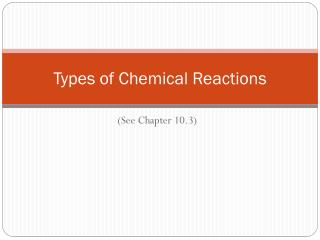DownloadDownload PresentationTypes of Chemical Reactions

# Types of Chemical Reactions

Download Presentation## Types of Chemical Reactions

- - - - - - - - - - - - - - - - - - - - - - - - - - - E N D - - - - - - - - - - - - - - - - - - - - - - - - - - -
##### Presentation Transcript

1. Types of Chemical Reactions (See Chapter 10.3)

2. Types of Chemical Reactions How many songs exist? Let’s say I want to sort my music. How could I do that?

3. Types of Chemical Reactions There are too many chemical equations to count. However, we can sort them into five general categories.

4. Type 1: Synthesis Reaction • Two compounds combine to make one compound A A + B B A + B AB

5. Type 1: Synthesis Reaction • Example O H H H O H + O O H H H H 2H2 + O2 2H2O

6. Type 2: Decomposition Reaction • One compound breaks apart into two or more compounds or elements A A B B + B AB A +

7. Type 2: Decomposition Reaction • Example O O Ca O C Ca C + O O O CaCO3(s) CaO(s) CO2(g) +

8. Type 3: Single Replacement Reaction • Two compounds swap a single element or polyatomic ion A A C C B B + + A B + + BC AC

9. Type 3: Single Replacement Reaction • Example Na Na Cl Cl K K + + Na(s) K(s) + + KCl(s) NaCl(s)

10. Type 4: Double Replacement Reaction • Two ions “trade partners” + + B A A C D C B D AB CB + + CD AD

11. Type 4: Double Replacement Reaction • Example K K I O O K H H H O I + + I Ca K Ca I O H 2KI(s) + Ca(OH)2(s) 2KOH(s) CaI2(s) +

12. Type 5: Combustion Reaction • A molecule reacts with O2 to make CO2, H2O, and lots of heat O O C + + C O O H H H O CxHy H2O + + CO2 O2

13. Type 5: Combustion Reaction • Example O H O O H C + + C O O O O H H H H H H O CH4 2H2O + + CO2 2O2

14. Practice • What type of reaction is: N2(g) + 3H2(g)  2NH3(g) 1) Synthesis 2) Decomposition 3) Single Replacement 4) Double Replacement 5) Combustion

15. Practice • What type of reaction is: NaNO3(s) + K(s) Na(s) + KNO3(s) 1) Synthesis 2) Decomposition 3) Single Replacement 4) Double Replacement 5) Combustion

16. Practice • What type of reaction is: 2C4H10(g) + 13O2(g) 8CO2(g) + 10H2O(g) 1) Synthesis 2) Decomposition 3) Single Replacement 4) Double Replacement 5) Combustion

17. Practice • What type of reaction is: 2H2O(g)  2H2(g) + O2(g) 1) Synthesis 2) Decomposition 3) Single Replacement 4) Double Replacement 5) Combustion

18. Practice • What type of reaction is: PbCl2(s) + 2HBr(g)  PbBr2(s) + 2HCl(g) 1) Synthesis 2) Decomposition 3) Single Replacement 4) Double Replacement 5) Combustion

19. Demonstration 1 Reactants: Products: • Chemical Equation (unbalanced) • Chemical Equation (balanced) • Type of Reaction C C12H22O11 H2O C12H22O11(s) C(s) + H2O(g) C12H22O11(s)  12C(s) +11 H2O(g) Decomposition

20. Demonstration 2 Reactants: Products: • Chemical Equation (unbalanced) • Chemical Equation (balanced) • Type of Reaction Mg O2 MgO Mg(s) + O2(g) MgO(s) 2Mg(s) + O2(g)  2MgO(s) Synthesis

21. Demonstration 3 Reactants: Products: • Chemical Equation (unbalanced) • Chemical Equation (balanced) • Type of Reaction Pb(NO3)2 NaOH Pb(OH)2 NaNO3 Pb(NO3)2(aq) + NaOH(aq)  Pb(OH)2(s) + NaNO3(aq) Pb(NO3)2(aq) + 2NaOH(aq)  Pb(OH)2(s) + 2NaNO3(aq) Double Replacement

22. Demonstration 4 Reactants: Products: • Chemical Equation (unbalanced) • Chemical Equation (balanced) • Type of Reaction Mg HCl MgCl2 H2 Mg(s) + HCl(aq)  MgCl2(aq) +H2(g) Mg(s) + 2HCl(aq)  MgCl2(aq) + H2(g) Single Replacement

23. Demonstration 5 Reactants: Products: • Chemical Equation (unbalanced) • Chemical Equation (balanced) • Type of Reaction CH4 O2 CO2 H2O CH4(g) + O2(g)  CO2(g) + H2O(g) CH4(g) + 2O2(g)  CO2(g) + 2H2O(g) Combustion